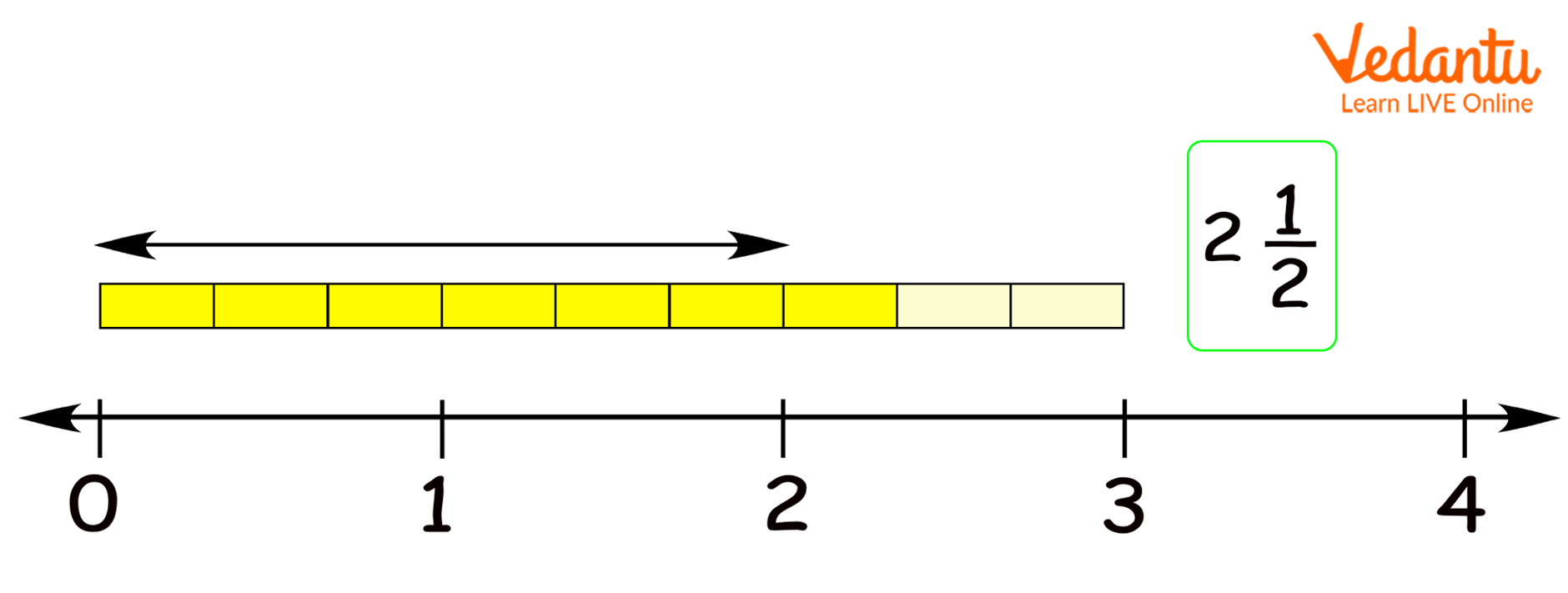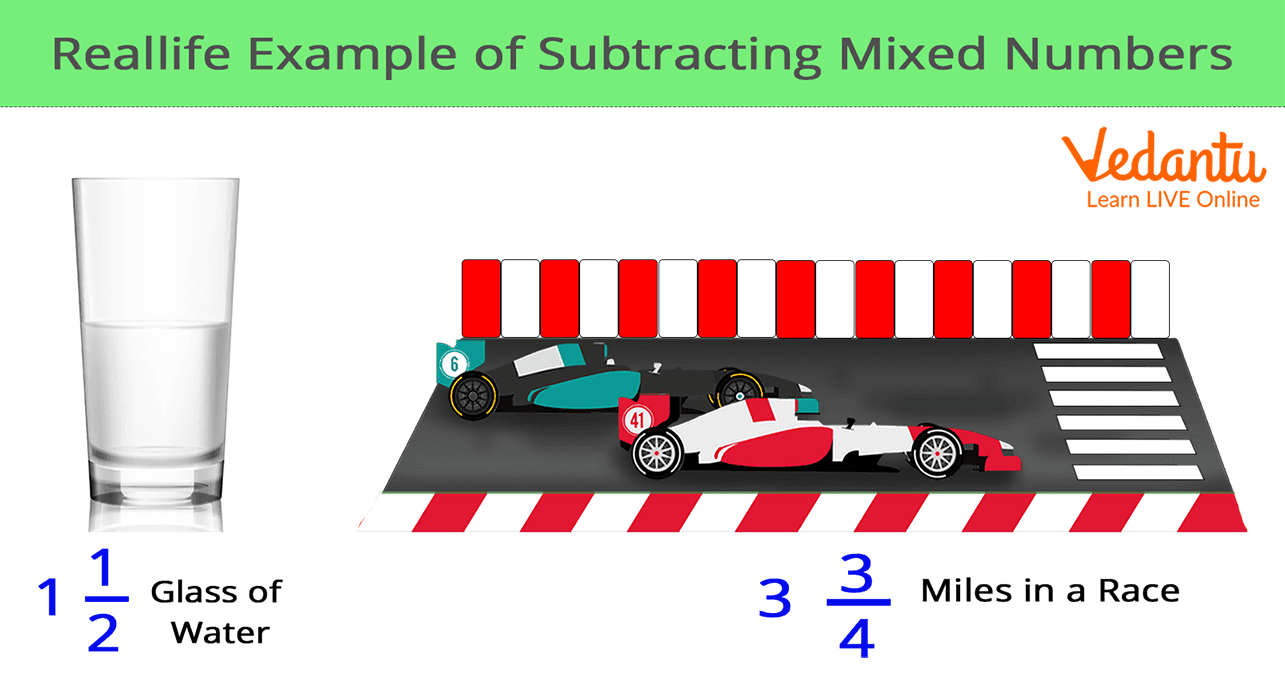Courses
Courses for Kids
Free study material
Free LIVE classes
More

# Subtraction of Mixed NumbersLIVE
Join Vedantu’s FREE Mastercalss

## What are Mixed Numbers? Learn About the Subtraction of These Numbers

Before we start our study on the topic of subtraction of mixed numbers, first let us get into the basics. What are Mixed Numbers? Mixed Numbers are the other name for mixed fractions. These are the fractions that are more than the whole number. Want to have more clarity on mixed fractions? Then refer to the example after this section.

Here we will talk about subtracting these mixed numbers or mixed fractions.

## Example of Mixed Numbers or Mixed Fractions

As already discussed, mixed numbers or mixed fractions are numbers that are more than just the whole numbers. Example: Renu has 3 chocolates, and each chocolate has 3 bars. She ate 7 bars of chocolate. This means that 2 full chocolate and 1 bar of the third chocolate she had eaten. This can be represented in the following diagram:Some real-life examples of mixed numbers are presented in the diagram given below:After gaining the basics of mixed numbers we will discuss the subtraction of these mixed numbers.

## How do you Subtract the Mixed Numbers?

We can subtract the mixed numbers in the same manner as to how we add the mixed numbers. Let us check the operation of subtracting mixed numbers step by step. In this, we will be dividing the steps into two cases. In Case I, we will talk about how to subtract the mixed numbers with the same denominator. In Case II, we will discuss the steps involved in the subtraction of the mixed numbers with different denominators.

Case 1: Subtracting mixed numbers with the same denominators.

Follow the simple steps to subtract the mixed numbers with the same denominators:

Step 1: First subtract the whole numbers.

Step 2: Now convert the fractions into the improper fractions.

Step 3: Now subtract the fractions.

Step 4: You can change the improper fraction into mixed numbers if required

Step 5: Now write the mixed numbers in whole and also the fractions.

Also, cite the example to understand the steps better

Example: 4 4⁄3 - 2 2⁄3

Step 1 - Subtract 4 from 2.

4 - 2 =2

Step 2 - Subtract the fractions:

4⁄3 - 2⁄3 = ⅔

Step 3 - Write the fraction with the whole.

4 4⁄3 - 2 2⁄3 = 2 2⁄3

Now, we will check Case 2 where the mixed numbers are subtracted with different denominators.

Case 2: Subtraction of the mixed numbers with different denominators.

Step 1: Firstly convert the mixed numbers with the different denominators.

Step 2: Now we have to find the common multiple of both the denominators.

Step 3: Convert the fractions as the common denominators.

Step 4: Now solve the fractions likewise.

Step 5: Convert the fractions into mixed numbers.

Let us check the example to understand with more clarity

Example 2: 6 1⁄2 - 1 3⁄4

Step 1 - Here we have to convert the mixed numbers into the form of improper fractions.

13⁄2 - 7⁄4

Step 2 - Then find the common multiple of both these denominators 2 and 4.

The Common multiple of 2 and 4 is 4.

Step 3 - Thereby convert the fractions as the common denominators.Step 4 - Now solve the fractions

26⁄4 - 7⁄4 = 19⁄4

Step 5 - After this convert the fraction into the mixed number form.

19⁄4 = 4 3⁄4

## Did You Know?

We can multiply a fraction by any number but the value of the fraction will always remain the same.

As we come to the end of our discussion, we hope now it is clear what are mixed fractions and you can thereby solve the mixed fractions if it is in the subtraction form. You can practice as many sums as you like to be clearer on this part.

Last updated date: 22nd Sep 2023
Total views: 139.5k
Views today: 4.39k

## FAQs on Subtraction of Mixed Numbers

1. How can we borrow while subtracting the mixed fractions?

In a mixed fraction, if the proper fractional part of the fraction where the other mixed fraction will be getting subtracted, the whole number there gives a borrow to the other proper fraction in order to make it large.

2. How do we convert the improper fraction into a mixed fraction?

Suppose, we want to convert the improper fraction into a mixed number, then we will divide the numerator of the fraction by the denominator of the fraction. After this division, the mixed number will be thus formed in a way that the quotient which is obtained will become the whole number while the remainder will become the new numerator and the denominator will be the same in this case.